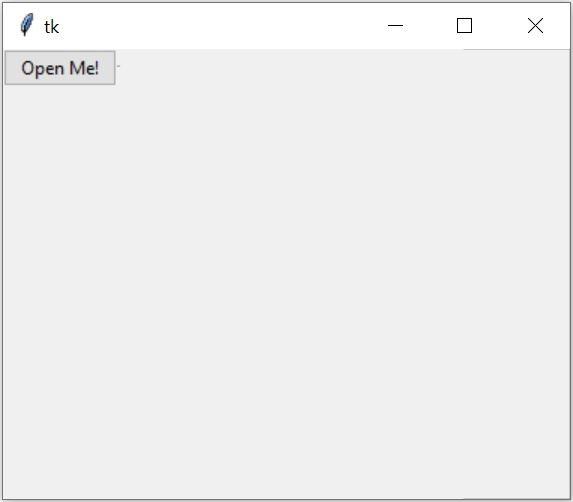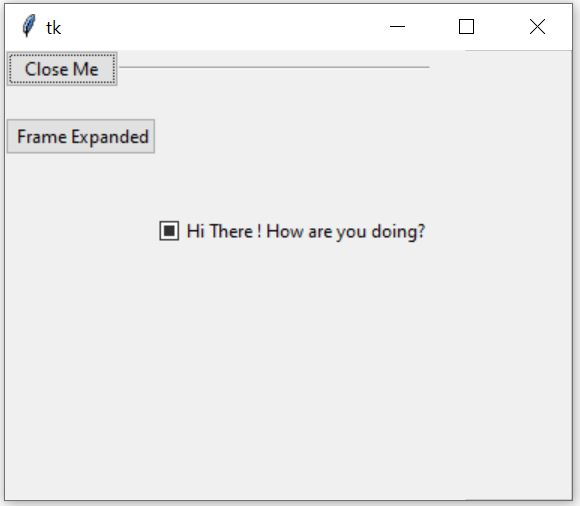# Collapsible Pane in Tkinter Python

Tkinter is the GUI building library of python. In this article we will see how we can create a collapsible pane. They are usefult when we have some large amount of data to be displayed over a GUI canvas but we do not want to be displayed always. It is made collapsible so that it can be displayed as and when needed.

The below program creates the collapsible pane where we see the result both after expanding and contracting the arrow. The code comments indicate the approach we take at each step.

## Example

from tkinter import *
import tkinter as tk
from tkinter import ttk
from tkinter.ttk import *
class cpane(ttk.Frame):
def __init__(self, MainWindow, expanded_text, collapsed_text):
ttk.Frame.__init__(self, MainWindow)
# The class variable
self.MainWindow = MainWindow
self._expanded_text = expanded_text
self._collapsed_text = collapsed_text
# Weight=1 to grow it's size as needed
self.columnconfigure(1, weight=1)
self._variable = tk.IntVar()
# Creating Checkbutton
self._button = ttk.Checkbutton(self, variable=self._variable,
command=self._activate, style="TButton")
self._button.grid(row=0, column=0)
# Create a Horizontal line
self._separator = ttk.Separator(self, orient="horizontal")
self._separator.grid(row=0, column=1, sticky="we")
self.frame = ttk.Frame(self)
# Activate the class
self._activate()
def _activate(self):
if not self._variable.get():
# Remove this widget when button pressed.
self.frame.grid_forget()
# Show collapsed text
self._button.configure(text=self._collapsed_text)
elif self._variable.get():
# Increase the frame area as needed
self.frame.grid(row=1, column=0, columnspan=2)
self._button.configure(text=self._expanded_text)
def toggle(self):
self._variable.set(not self._variable.get())
self._activate()
# Creating root window or MainWindow
root = Tk()
root.geometry('300x300')
# Creating Object of Collapsible Pane Container
cpane_obj = cpane(root, 'Close Me', 'Open Me!')
cpane_obj.grid(row=0, column=0)
# Buttons to # appear in collapsible pane
b = Button(cpane_obj.frame, text=" Frame Expanded").grid(
b = Checkbutton(cpane_obj.frame, text="Hi There ! How are you doing?").grid(
mainloop()

## Output

Running the above code gives us the following result −Updated on: 18-Feb-2020

563 Views